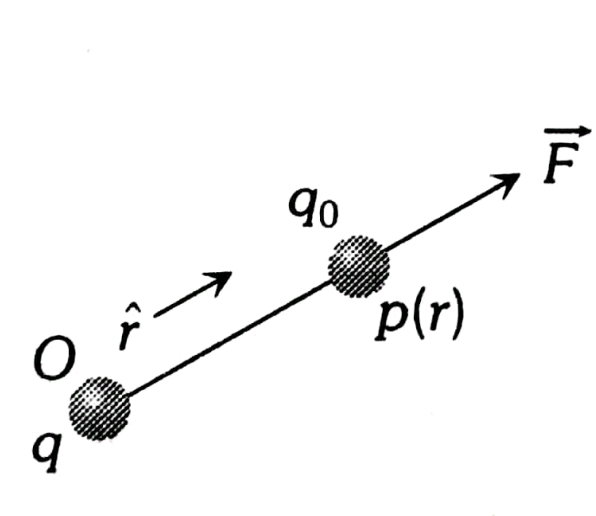Q

# Confused! kindly explain, - Electrostatics - JEE Main-7

The distance between the two charges   and    is 11 cm  At what point on the line joining the two, the intensity will be zero

• Option 1)

At a distance of 5 cm from

• Option 2)

At a distance of 5 cm from

• Option 3)

At a distance of 10 cm from

• Option 4)

At a distance of 11 cm from

78 Views

As we learned

Electric Field Intensity -

- whereinSuppose electric field is zero at point N in the figure thenAt N |E1| = |E2 | which gives

Option 1)

At a distance of 5 cm from

Option 2)

At a distance of 5 cm from

Option 3)

At a distance of 10 cm from

Option 4)

At a distance of 11 cm from

Exams
Articles
Questions